### Learning Objectives

• Analyze a function and its derivatives to draw its graph.

We have shown how to use the first and second derivatives of a function to describe the shape of a graph. Now we put everything together with other features to graph a function $f$.

# Guidelines for Drawing the Graph of a Function

We now have enough analytical tools to draw graphs of a wide variety of algebraic and transcendental functions. Before showing how to graph specific functions, let’s look at a general strategy to use when graphing any function.

### Problem-Solving Strategy: Drawing the Graph of a Function

Given a function $f,$ use the following steps to sketch a graph of $f\text{:}$

1. Determine the domain of the function.
2. Locate the $x$– and $y$-intercepts.
3. Evaluate $\underset{x\to \infty }{\text{lim}}f(x)$ and $\underset{x\to -\infty }{\text{lim}}f(x)$ to determine horizontal or oblique asymptote .
4. Determine whether $f$ has any vertical asymptotes.
5. Calculate ${f}^{\prime }.$ Find all critical numbers and determine the intervals where $f$ is increasing and where $f$ is decreasing. Determine whether $f$ has any local extrema.
6. Calculate $f''(x).$ Determine the intervals where $f$ is concave up and where $f$ is concave down. Use this information to determine whether $f$ has any inflection points. The second derivative can also be used as an alternate means to determine or verify that $f$ has a local extremum at a critical number.

Now let’s use this strategy to graph several different functions. We start by graphing a polynomial function.

### Sketching a Graph of a Polynomial

Sketch a graph of $f(x)={(x-1)}^{2}(x+2).$

#### Solution

Step 1. Since $f$ is a polynomial, the domain is the set of all real numbers.

Step 2. When $x=0,f(x)=2.$ Therefore, the $y$-intercept is $(0,2).$ To find the $x$-intercepts, we need to solve the equation ${(x-1)}^{2}(x+2)=0,$ gives us the $x$-intercepts $(1,0)$ and $(-2,0)$

Step 3. We need to evaluate the end behavior of $f.$ As $x\to \infty ,$ ${(x-1)}^{2}\to \infty$ and $(x+2)\to \infty .$ Therefore, $\underset{x\to \infty }{\text{lim}}f(x)=\infty .$ As $x\to -\infty ,$ ${(x-1)}^{2}\to \infty$ and $(x+2)\to -\infty .$ Therefore, $\underset{x\to \infty }{\text{lim}}f(x)=-\infty .$

Step 4. Since $f$ is a polynomial function, it does not have any vertical asymptotes.

Step 5. The first derivative of $f$ is

${f}^{\prime }(x)=3{x}^{2}-3.$

Therefore, $f$ has two critical numbers: $x=1,-1.$ Divide the interval $(-\infty ,\infty )$ into the three smaller intervals: $(-\infty ,-1),$ $(-1,1),$ and $(1,\infty ).$ Then, choose test points $x=-2,$ $x=0,$ and $x=2$ from these intervals and evaluate the sign of ${f}^{\prime }(x)$ at each of these test points, as shown in the following table.

Interval Test Point Sign of Derivative ${f}^{\prime } (x)=3{x}^{2}-3=3(x-1)(x+1)$ Conclusion
$(-\infty ,-1)$ $x=-2$ $(\text{+})(-)(-)=+$ $f$ is increasing.
$(-1,1)$ $x=0$ $(\text{+})(-)(\text{+})=-$ $f$ is decreasing.
$(1,\infty )$ $x=2$ $(\text{+})(\text{+})(\text{+})=+$ $f$ is increasing.

From the table, we see that $f$ has a local maximum at $x=-1$ and a local minimum at $x=1.$ Evaluating $f(x)$ at those two points, we find that the local maximum value is $f(-1)=4$ and the local minimum value is $f(1)=0.$

Step 6. The second derivative of $f$ is

$f''(x)=6x.$

The second derivative is zero at $x=0.$ Therefore, to determine the concavity of $f,$ divide the interval $(-\infty ,\infty )$ into the smaller intervals $(-\infty ,0)$ and $(0,\infty ),$ and choose test points $x=-1$ and $x=1$ to determine the concavity of $f$ on each of these smaller intervals as shown in the following table.

Interval Test Point Sign of $f''(x)=6x$ Conclusion
$(-\infty ,0)$ $x=-1$ $-$ $f$ is concave down.
$(0,\infty )$ $x=1$ $+$ $f$ is concave up.

We note that the information in the preceding table confirms the fact, found in step 5, that $f$ has a local maximum at $x=-1$ and a local minimum at $x=1.$ In addition, the information found in step 5—namely, $f$ has a local maximum at $x=-1$ and a local minimum at $x=1,$ and ${f}^{\prime }(x)=0$ at those points—combined with the fact that $f''(x)$ changes sign only at $x=0$ confirms the results found in step 6 on the concavity of $f.$

Combining this information, we arrive at the graph of $f(x)={(x-1)}^{2}(x+2)$ shown in the following graph.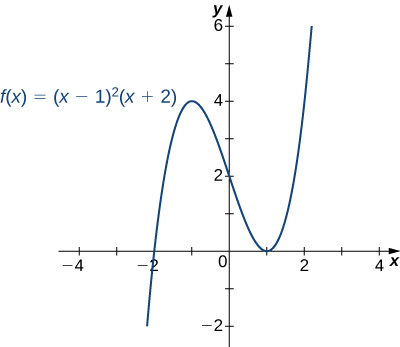Sketch a graph of $f(x)={(x-1)}^{3}(x+2).$

#### Solution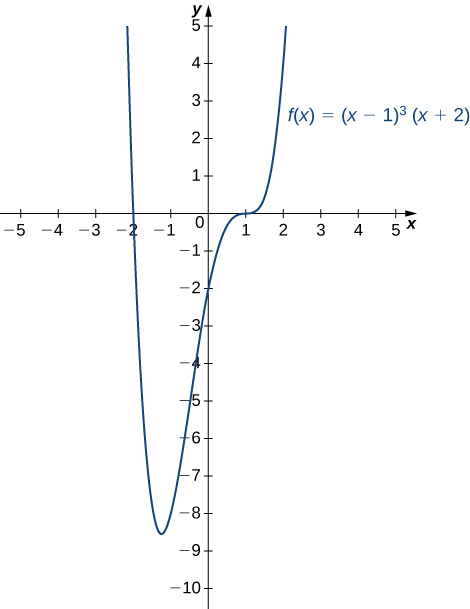### Sketching a Rational Function

Sketch the graph of $f(x)=\frac{{x}^{2}}{(1-{x}^{2})}\text{.}$

#### Solution

Step 1. The function $f$ is defined as long as the denominator is not zero. Therefore, the domain is the set of all real numbers $x$ except $x=\pm 1.$

Step 2. Find the intercepts. If $x=0,$ then $f(x)=0,$ so 0 is an intercept. If $y=0,$ then $\frac{{x}^{2}}{(1-{x}^{2})}=0,$ which implies $x=0.$ Therefore, $(0,0)$ is the only intercept.

Step 3. Evaluate the limits at infinity. Since $f$ is a rational function, divide the numerator and denominator by the highest power in the denominator: ${x}^{2}.$ We obtain

$\underset{x\to \pm \infty }{\text{lim}}\frac{{x}^{2}}{1-{x}^{2}}=\underset{x\to \pm \infty }{\text{lim}}\frac{1}{\frac{1}{{x}^{2}}-1}=-1.$

Therefore, $f$ has a horizontal asymptote of $y=-1$ as $x\to \infty$ and $x\to -\infty .$

Step 4. To determine whether $f$ has any vertical asymptotes, first check to see whether the denominator has any zeroes. We find the denominator is zero when $x=\pm 1.$ To determine whether the lines $x=1$ or $x=-1$ are vertical asymptotes of $f,$ evaluate $\underset{x\to 1}{\text{lim}}f(x)$ and $\underset{x\to -1}{\text{lim}}f(x).$ By looking at each one-sided limit as $x\to 1,$ we see that

$\underset{x\to {1}^{+}}{\text{lim}}\frac{{x}^{2}}{1-{x}^{2}}=-\infty \text{ and }\underset{x\to {1}^{-}}{\text{lim}}\frac{{x}^{2}}{1-{x}^{2}}=\infty .$

In addition, by looking at each one-sided limit as $x\to -1,$ we find that

$\underset{x\to -{1}^{+}}{\text{lim}}\frac{{x}^{2}}{1-{x}^{2}}=\infty \text{ and }\underset{x\to -{1}^{-}}{\text{lim}}\frac{{x}^{2}}{1-{x}^{2}}=-\infty .$

Step 5. Calculate the first derivative:

${f}^{\prime }(x)=\frac{(1-{x}^{2})(2x)-{x}^{2}(-2x)}{{(1-{x}^{2})}^{2}}=\frac{2x}{{(1-{x}^{2})}^{2}}.$

Critical numbers occur at points $x$ where ${f}^{\prime }(x)=0$ or ${f}^{\prime }(x)$ is undefined. We see that ${f}^{\prime }(x)=0$ when $x=0.$ The derivative ${f}^{\prime }$ is not undefined at any point in the domain of $f.$ However, $x=\pm 1$ are not in the domain of $f.$ Therefore, to determine where $f$ is increasing and where $f$ is decreasing, divide the interval $(-\infty ,\infty )$ into four smaller intervals: $(-\infty ,-1),$ $(-1,0),$ $(0,1),$ and $(1,\infty ),$ and choose a test point in each interval to determine the sign of ${f}^{\prime }(x)$ in each of these intervals. The values $x=-2,$ $x=-\frac{1}{2},$ $x=\frac{1}{2},$ and $x=2$ are good choices for test points as shown in the following table.

Interval Test Point Sign of ${f}^{\prime }(x)=\frac{2x}{{(1-{x}^{2})}^{2}}$ Conclusion
$(-\infty ,-1)$ $x=-2$ $-\text{/}+=-$ $f$ is decreasing.
$(-1,0)$ $x=-1\text{/}2$ $-\text{/}+=-$ $f$ is decreasing.
$(0,1)$ $x=1\text{/}2$ $+\text{/}+=+$ $f$ is increasing.
$(1,\infty )$ $x=2$ $+\text{/}+=+$ $f$ is increasing.

From this analysis, we conclude that $f$ has a local minimum at $x=0$ but no local maximum.

Step 6. Calculate the second derivative:

$\begin{array}{cc}\hfill f''(x)& \hfill =\frac{{(1-{x}^{2})}^{2}(2)-2x(2(1-{x}^{2})(-2x))}{{(1-{x}^{2})}^{4}}\\ & =\frac{(1-{x}^{2})\left[2(1-{x}^{2})+8{x}^{2}\right]}{{(1-{x}^{2})}^{4}}\hfill \\ & =\frac{2(1-{x}^{2})+8{x}^{2}}{{(1-{x}^{2})}^{3}}\hfill \\ & =\frac{6{x}^{2}+2}{{(1-{x}^{2})}^{3}}.\hfill \end{array}$

To determine the intervals where $f$ is concave up and where $f$ is concave down, we first need to find all points $x$ where $f''(x)=0$ or $f''(x)$ is undefined. Since the numerator $6{x}^{2}+2\ne 0$ for any $x,$ $f''(x)$ is never zero. Furthermore, $f''(x)$ is not undefined for any $x$ in the domain of $f.$ However, as discussed earlier, $x=\pm 1$ are not in the domain of $f.$ Therefore, to determine the concavity of $f,$ we divide the interval $(-\infty ,\infty )$ into the three smaller intervals $(-\infty ,-1),$ $(-1,-1),$ and $(1,\infty ),$ and choose a test point in each of these intervals to evaluate the sign of $f''(x).$ in each of these intervals. The values $x=-2,$ $x=0,$ and $x=2$ are possible test points as shown in the following table.

Interval Test Point Sign of $f''(x)=\frac{6{x}^{2}+2}{{(1-{x}^{2})}^{3}}$ Conclusion
$(-\infty ,-1)$ $x=-2$ $+\text{/}-=-$ $f$ is concave down.
$(-1,-1)$ $x=0$ $+\text{/}+=+$ $f$ is concave up.
$(1,\infty )$ $x=2$ $+\text{/}-=-$ $f$ is concave down.

Combining all this information, we arrive at the graph of $f$ shown below. Note that, although $f$ changes concavity at $x=-1$ and $x=1,$ there are no inflection points at either of these places because $f$ is not continuous at $x=-1$ or $x=1.$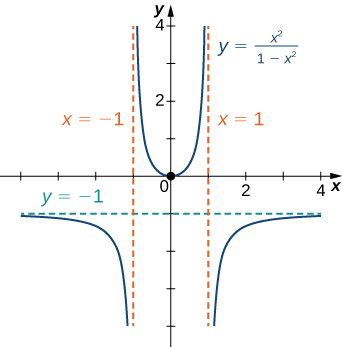Sketch a graph of $f(x)=\frac{(3x+5)}{(8+4x)}.$

#### Hint

A line $y=L$ is a horizontal asymptote of $f$ if the limit as $x\to \infty$ or the limit as $x\to -\infty$ of $f(x)$ is $L.$ A line $x=a$ is a vertical asymptote if at least one of the one-sided limits of $f$ as $x\to a$ is $\infty$ or $-\infty .$

#### Solution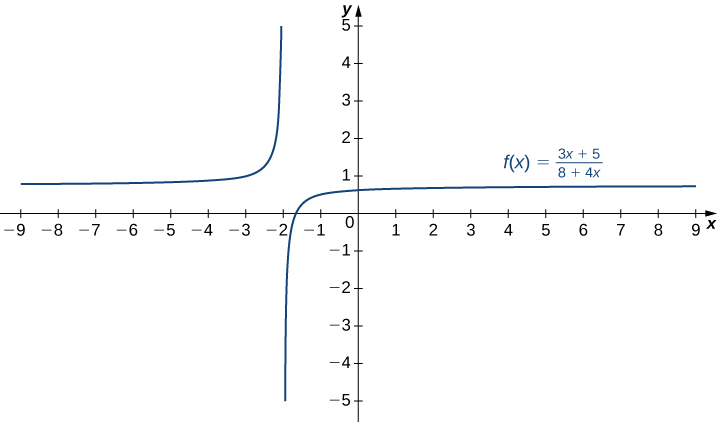### Sketching a Rational Function with an Oblique Asymptote

Sketch the graph of $f(x)=\frac{{x}^{2}}{(x-1)}$

#### Solution

Step 1. The domain of $f$ is the set of all real numbers $x$ except $x=1.$

Step 2. Find the intercepts. We can see that when $x=0,$ $f(x)=0,$ so $(0,0)$ is the only intercept.

Step 3. Evaluate the limits at infinity. Since the degree of the numerator is one more than the degree of the denominator, $f$ must have an oblique asymptote. To find the oblique asymptote, use long division of polynomials to write

$f(x)=\frac{{x}^{2}}{x-1}=x+1+\frac{1}{x-1}.$

Since $1\text{/}(x-1)\to 0$ as $x\to \pm \infty ,$ $f(x)$ approaches the line $y=x+1$ as $x\to \pm \infty .$ The line $y=x+1$ is an oblique asymptote for $f.$

Step 4. To check for vertical asymptotes, look at where the denominator is zero. Here the denominator is zero at $x=1.$ Looking at both one-sided limits as $x\to 1,$ we find

$\underset{x\to {1}^{+}}{\text{lim}}\frac{{x}^{2}}{x-1}=\infty \text{ and }\underset{x\to {1}^{-}}{\text{lim}}\frac{{x}^{2}}{x-1}=-\infty .$

Therefore, $x=1$ is a vertical asymptote, and we have determined the behavior of $f$ as $x$ approaches 1 from the right and the left.

Step 5. Calculate the first derivative:

${f}^{\prime }(x)=\frac{(x-1)(2x)-{x}^{2}(1)}{{(x-1)}^{2}}=\frac{{x}^{2}-2x}{{(x-1)}^{2}}.$

We have ${f}^{\prime }(x)=0$ when ${x}^{2}-2x=x(x-2)=0.$ Therefore, $x=0$ and $x=2$ are critical numbers. Since $f$ is undefined at $x=1,$ we need to divide the interval $(-\infty ,\infty )$ into the smaller intervals $(-\infty ,0),$ $(0,1),$ $(1,2),$ and $(2,\infty ),$ and choose a test point from each interval to evaluate the sign of ${f}^{\prime }(x)$ in each of these smaller intervals. For example, let $x=-1,$ $x=\frac{1}{2},$ $x=\frac{3}{2},$ and $x=3$ be the test points as shown in the following table.

Interval Test Point Sign of ${f}^{\prime } (x)=\frac{{x}^{2}-2x}{{(x-1)}^{2}}=\frac{x(x-2)}{{(x-1)}^{2}}$ Conclusion
$(-\infty ,0)$ $x=-1$ $(-)(-)\text{/}+=+$ $f$ is increasing.
$(0,1)$ $x=1\text{/}2$ $(\text{+})(-)\text{/}+=-$ $f$ is decreasing.
$(1,2)$ $x=3\text{/}2$ $(\text{+})(-)\text{/}+=-$ $f$ is decreasing.
$(2,\infty )$ $x=3$ $(\text{+})(\text{+})\text{/}+=+$ $f$ is increasing.

From this table, we see that $f$ has a local maximum at $x=0$ and a local minimum at $x=2.$ The value of $f$ at the local maximum is $f(0)=0$ and the value of $f$ at the local minimum is $f(2)=4.$ Therefore, $(0,0)$ and $(2,4)$ are important points on the graph.

Step 6. Calculate the second derivative:

$\begin{array}{cc}\hfill f''(x)& =\frac{{(x-1)}^{2}(2x-2)-({x}^{2}-2x)(2(x-1))}{{(x-1)}^{4}}\hfill \\ & =\frac{(x-1)\left[(x-1)(2x-2)-2({x}^{2}-2x)\right]}{{(x-1)}^{4}}\hfill \\ & =\frac{(x-1)(2x-2)-2({x}^{2}-2x)}{{(x-1)}^{3}}\hfill \\ & =\frac{2{x}^{2}-4x+2-(2{x}^{2}-4x)}{{(x-1)}^{3}}\hfill \\ & =\frac{2}{{(x-1)}^{3}}.\hfill \end{array}$

We see that $f''(x)$ is never zero or undefined for $x$ in the domain of $f.$ Since $f$ is undefined at $x=1,$ to check concavity we just divide the interval $(-\infty ,\infty )$ into the two smaller intervals $(-\infty ,1)$ and $(1,\infty ),$ and choose a test point from each interval to evaluate the sign of $f''(x)$ in each of these intervals. The values $x=0$ and $x=2$ are possible test points as shown in the following table.

Interval Test Point Sign of $f''(x)=\frac{2}{{(x-1)}^{3}}$ Conclusion
$(-\infty ,1)$ $x=0$ $+\text{/}-=-$ $f$ is concave down.
$(1,\infty )$ $x=2$ $+\text{/}+=+$ $f$ is concave up.

From the information gathered, we arrive at the following graph for $f.$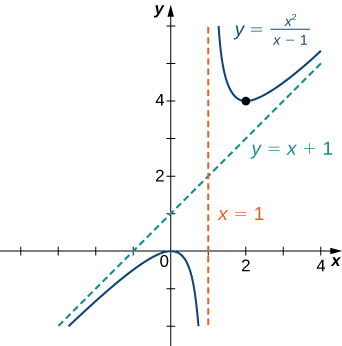Find the oblique asymptote for $f(x)=\frac{(3{x}^{3}-2x+1)}{(2{x}^{2}-4)}.$

#### Hint

Use long division of polynomials.

#### Solution

$y=\frac{3}{2}x$

### Sketching the Graph of a Function with a Cusp

Sketch a graph of $f(x)={(x-1)}^{2\text{/}3}.$

#### Solution

Step 1. Since the cube-root function is defined for all real numbers $x$ and ${(x-1)}^{2\text{/}3}={(\sqrt{x-1})}^{2},$ the domain of $f$ is all real numbers.

Step 2: To find the $y$-intercept, evaluate $f(0).$ Since $f(0)=1,$ the $y$-intercept is $(0,1).$ To find the $x$-intercept, solve ${(x-1)}^{2\text{/}3}=0.$ The solution of this equation is $x=1,$ so the $x$-intercept is $(1,0).$

Step 3: Since $\underset{x\to \pm \infty }{\text{lim}}{(x-1)}^{2\text{/}3}=\infty ,$ the function continues to grow without bound as $x\to \infty$ and $x\to -\infty .$

Step 4: The function has no vertical asymptotes.

Step 5: To determine where $f$ is increasing or decreasing, calculate ${f}^{\prime }.$ We find

${f}^{\prime }(x)=\frac{2}{3}{(x-1)}^{-1/3}=\frac{2}{3{(x-1)}^{1/3}}.$

This function is not zero anywhere, but it is undefined when $x=1.$ Therefore, the only critical number is $x=1.$ Divide the interval $(-\infty ,\infty )$ into the smaller intervals $(-\infty ,1)$ and $(1,\infty ),$ and choose test points in each of these intervals to determine the sign of ${f}^{\prime }(x)$ in each of these smaller intervals. Let $x=0$ and $x=2$ be the test points as shown in the following table.

Interval Test Point Sign of ${f}^{\prime }(x)=\frac{2}{3{(x-1)}^{1\text{/}3}}$ Conclusion
$(-\infty ,1)$ $x=0$ $+\text{/}-=-$ $f$ is decreasing.
$(1,\infty )$ $x=2$ $+\text{/}+=+$ $f$ is increasing.

We conclude that $f$ has a local minimum at $x=1.$ Evaluating $f$ at $x=1,$ we find that the value of $f$ at the local minimum is zero. Note that ${f}^{\prime }(1)$ is undefined, so to determine the behavior of the function at this critical number, we need to examine $\underset{x\to 1}{\text{lim}}{f}^{\prime }(x).$ Looking at the one-sided limits, we have

$\underset{x\to {1}^{+}}{\text{lim}}\frac{2}{3{(x-1)}^{1\text{/}3}}=\infty \text{ and }\underset{x\to {1}^{-}}{\text{lim}}\frac{2}{3{(x-1)}^{1\text{/}3}}=-\infty .$

Therefore, $f$ has a cusp at $x=1.$

Step 6: To determine concavity, we calculate the second derivative of $f\text{:}$

$f''(x)=-\frac{2}{9}{(x-1)}^{-4\text{/}3}=\frac{-2}{9{(x-1)}^{4\text{/}3}}.$

We find that $f''(x)$ is defined for all $x,$ but is undefined when $x=1.$ Therefore, divide the interval $(-\infty ,\infty )$ into the smaller intervals $(-\infty ,1)$ and $(1,\infty ),$ and choose test points to evaluate the sign of $f''(x)$ in each of these intervals. As we did earlier, let $x=0$ and $x=2$ be test points as shown in the following table.

Interval Test Point Sign of $f''(x)=\frac{-2}{9{(x-1)}^{4\text{/}3}}$ Conclusion
$(-\infty ,1)$ $x=0$ $-\text{/}+=-$ $f$ is concave down.
$(1,\infty )$ $x=2$ $-\text{/}+=-$ $f$ is concave down.

From this table, we conclude that $f$ is concave down everywhere. Combining all of this information, we arrive at the following graph for $f.$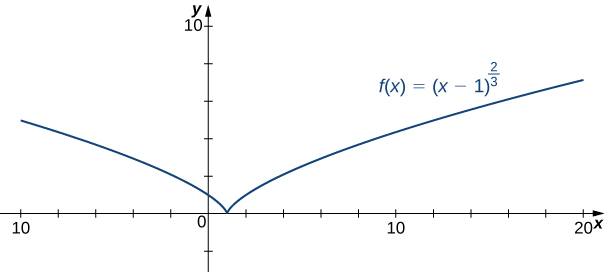Consider the function $f(x)=5-{x}^{2\text{/}3}.$ Determine the point on the graph where a cusp is located. Determine the end behavior of $f.$

#### Hint

A function $f$ has a cusp at a point $a$ if $f(a)$ exists, ${f}^{\prime } (a)$ is undefined, one of the one-sided limits as $x\to a$ of ${f}^{\prime } (x)$ is $+\infty ,$ and the other one-sided limit is $-\infty .$

#### Solution

The function $f$ has a cusp at $(0,5)$ $\underset{x\to {0}^{-}}{\text{lim}}{f}^{\prime }(x)=\infty ,$ $\underset{x\to {0}^{+}}{\text{lim}}{f}^{\prime }(x)=-\infty .$ For end behavior, $\underset{x\to \pm \infty }{\text{lim}}f(x)=-\infty .$

For the following exercises, sketch the function by finding the following:

1. Determine the domain of the function.
2. Determine the $x$– and $y$-intercepts.
3. Determine any horizontal or vertical asymptotes.
4. Determine the intervals where the function is increasing and where the function is decreasing. Determine whether the function has any local extrema.
5. Determine the intervals where the function is concave up and where the function is concave down.
6. Determine all inflection points (if any).

1. $y=3{x}^{2}+2x+4$

2. $y={x}^{3}-3{x}^{2}+4$

#### Solution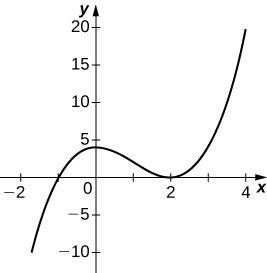3. $y=\frac{2x+1}{{x}^{2}+6x+5}$

4. $y=\frac{{x}^{3}+4{x}^{2}+3x}{3x+9}$

#### Solution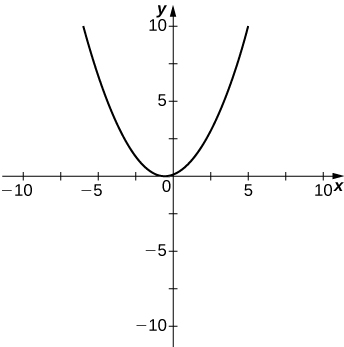5. $y=\frac{{x}^{2}+x-2}{{x}^{2}-3x-4}$

6. $y=\sqrt{{x}^{2}-5x+4}$

#### Solution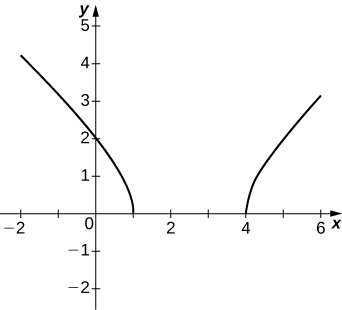7. $y=2x\sqrt{16-{x}^{2}}$

8. $y=\frac{ \cos x}{x},$ on $x=\left[-2\pi ,2\pi \right]$

#### Solution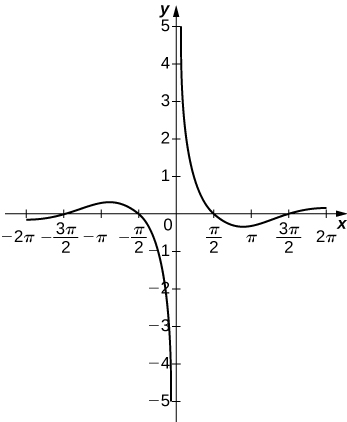9. $y={e}^{x}-{x}^{3}$

10. $y=x \tan x,x=\left[-\pi ,\pi \right]$

#### Solution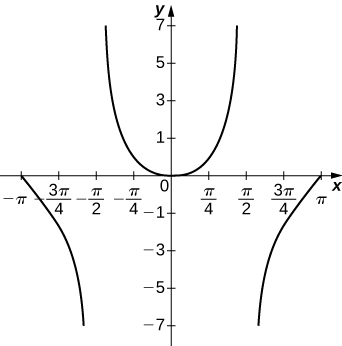11. $y=x\text{ln}(x),x < 0$

12. $y={x}^{2} \sin (x),x=\left[-2\pi ,2\pi \right]$

#### Solution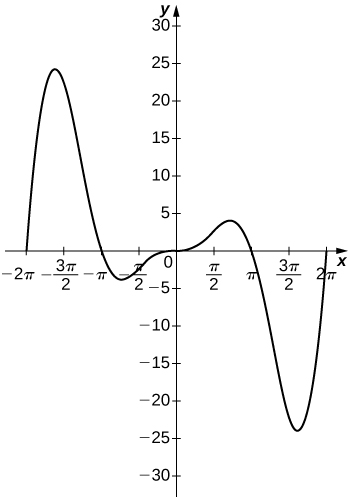13. $y = 2x + e^{-x}$

For the following exercises, sketch the graph of $f$ by finding the following:

1. Determine the domain of the function.
2. Determine the $x$– and $y$-intercepts.
3. Determine any horizontal or vertical asymptotes.
4. Determine the intervals where $f$ is increasing and where $f$ is decreasing. Determine whether $f$ has any local extrema.
5. Determine the intervals where $f$ is concave up and where $f$ is concave down.
6. Determine all inflection points (if any).

14. $f(x) = \frac{2x^2 -1}{(x-3)^2}, f'(x) = \frac{-12x+2}{(x-3)^3}, f''(x) = \frac{24x+30}{(x-3)^4}$

#### Solution15. $f(x) = \frac{x^3}{x-4}, f'(x) = \frac{2x^3-12x}{(x-4)^2}, f''(x) = \frac{2x^3-24x^2+96x}{(x-4)^3}$

16. $f(x) = 2\ln(1+x^2), f'(x) = \frac{4x}{1+x^2}, f''(x) = \frac{4(1-x^2)}{(1+x^2)^2}$

#### Solution17. $f(x) = \frac{e^{2x}}{x}, f'(x) = \frac{2e^{2x}-e^{2x}}{x^2}, f''(x) = \frac{2e^{2x}(2x^2-2x+1)}{x^3}$